# IB DP Physics: SL复习笔记2.1.7 Projectile Motion

### Projectile Motion

• The trajectory of an object undergoing projectile motion consists of a vertical component and a horizontal component
• These need to be evaluated separately
• Some key terms to know, and how to calculate them, are:
• Time of flight: how long the projectile is in the air
• Maximum height attained: the height at which the projectile is momentarily at rest
• Range: the horizontal distance traveled by the projectile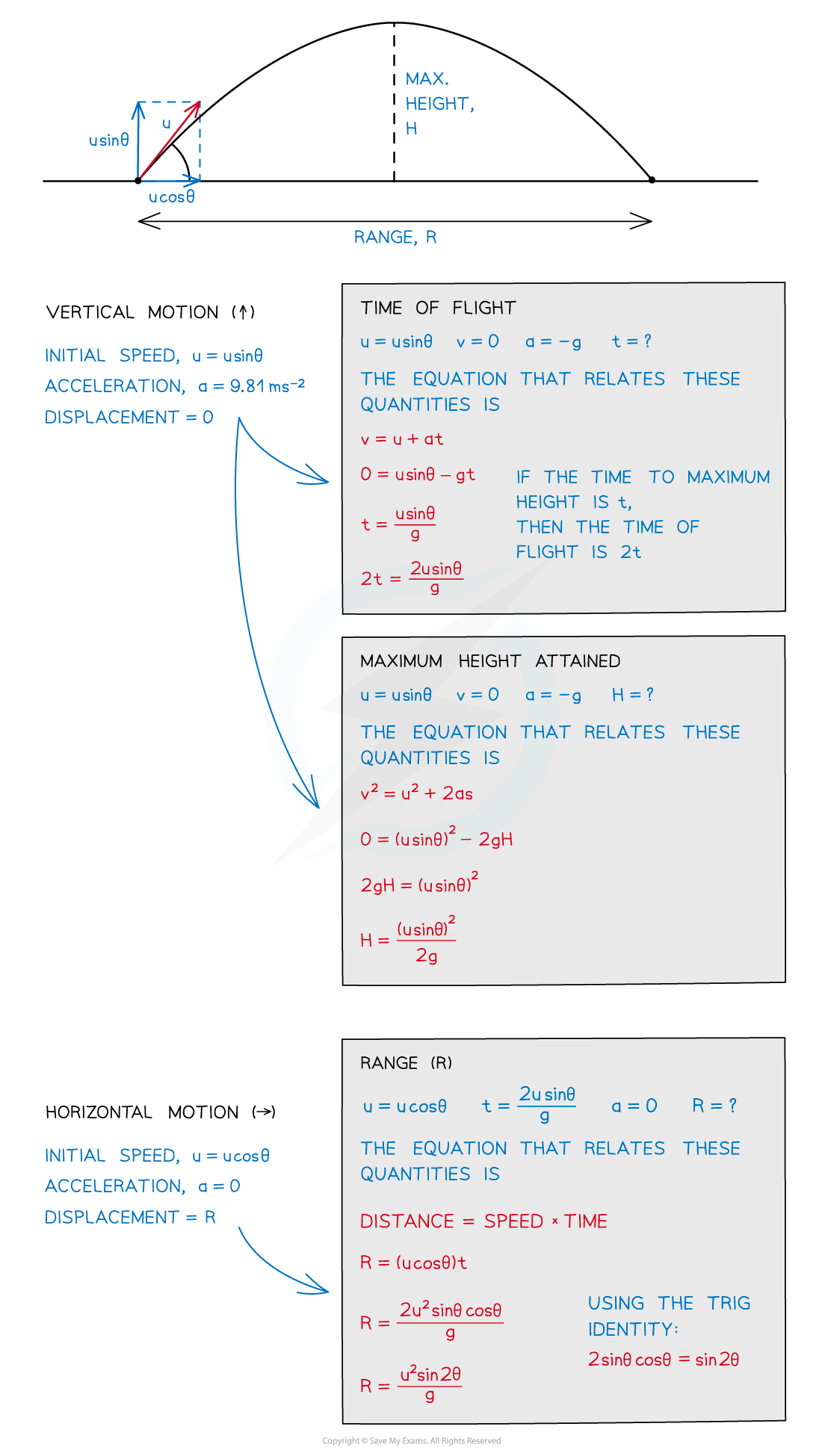How to find the time of flight, maximum height and range

#### Problems involving projectile motion

• There are two main considerations for solving problems involving two-dimensional motion of a projectile
• Constant velocity in the horizontal direction
• Constant acceleration in a perpendicular direction
• The only force acting on the projectile, after it has been released, is gravity
• There are three possible scenarios for projectile motion:
• Vertical projection
• Horizontal projection
• Projection at an angle

#### To calculate vertical projection (free fall)

A science museum designed an experiment to show the fall of a feather in a vertical glass vacuum tube.

The time of fall from rest is 0.5 s.What is the length of the tube, L?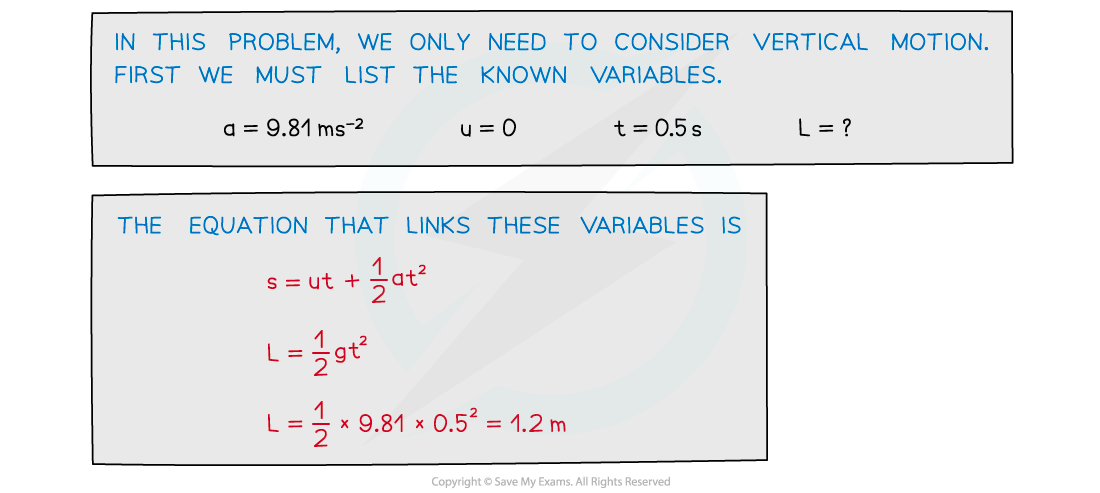#### To calculate horizontal projection

A motorcycle stunt-rider moving horizontally takes off from a point 1.25 m above the ground, landing 10 m away as shown.

What was the speed at take-off? (ignoring air resistance)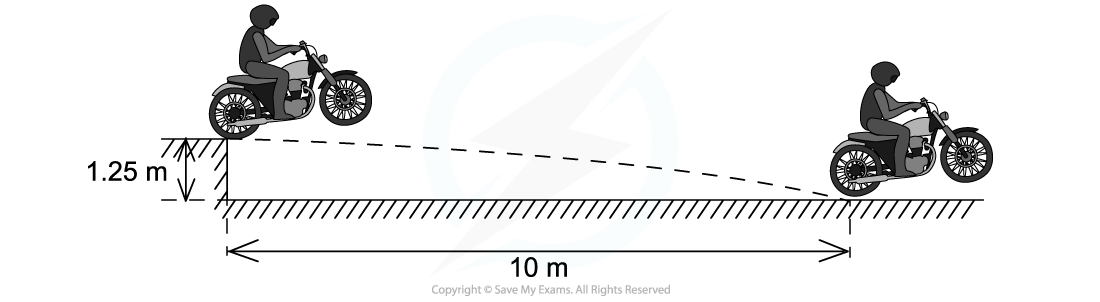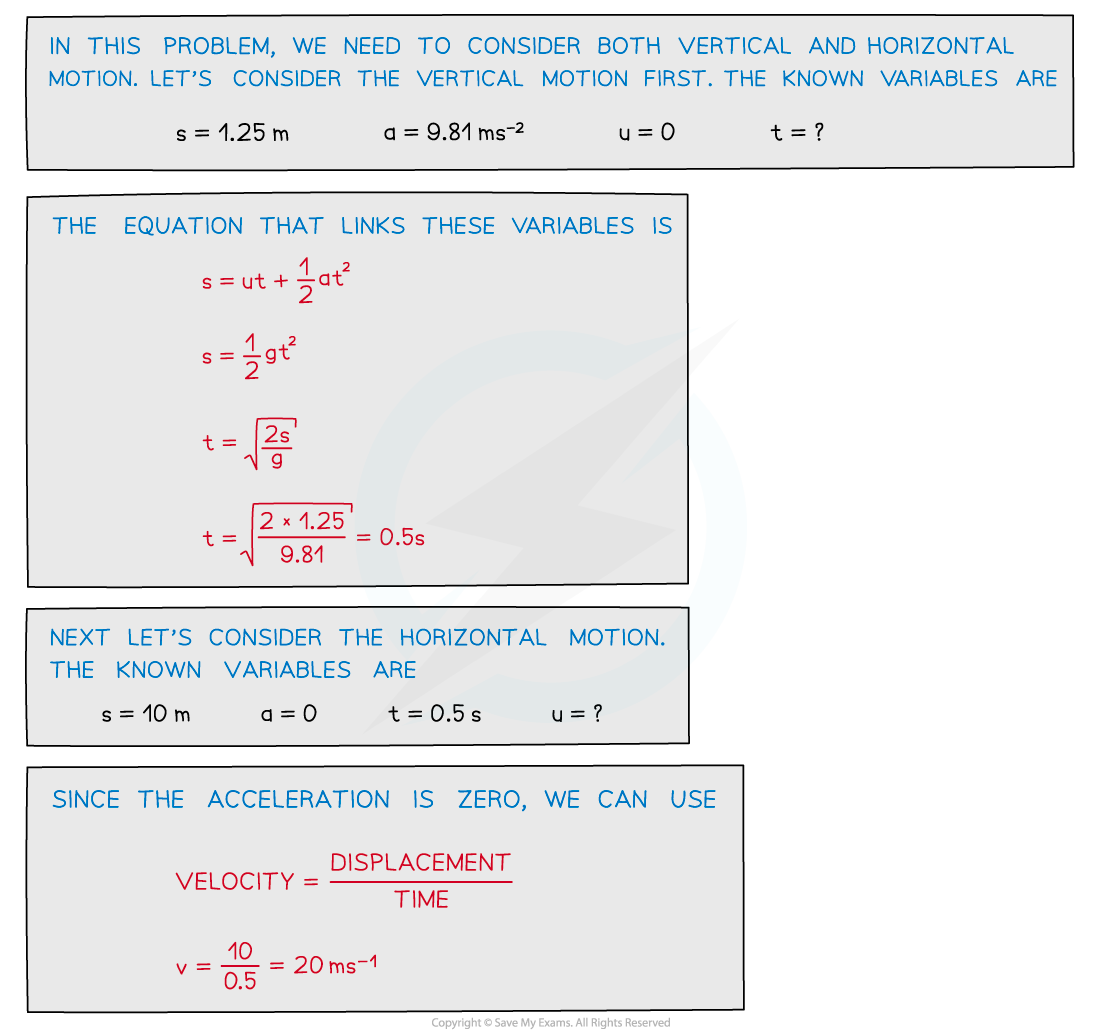#### To calculate projection at an angle

A ball is thrown from a point P with an initial velocity u of 12 m s-1 at 50° to the horizontal.

What is the value of the maximum height at Q? (ignoring air resistance)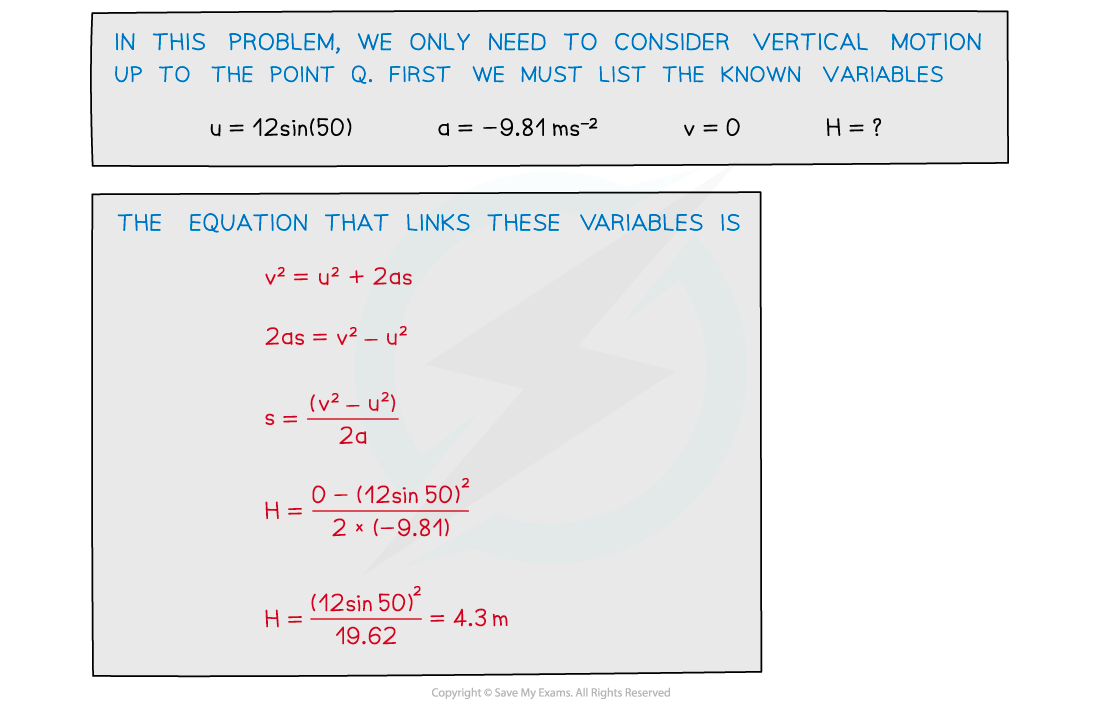#### Exam Tip

Make sure you don’t make these common mistakes:

• Forgetting that deceleration is negative as the object rises
• Confusing the direction of sin θ and cos θ
• Not converting units (mm, cm, km etc.) to metres

Further, it is worth noting that projectile motion is typically symmetrical when air resistance is ignored allowing for use of the peak to find the time of total flight or total horizontal distance by doubling the amount to get from the start point to the peak.